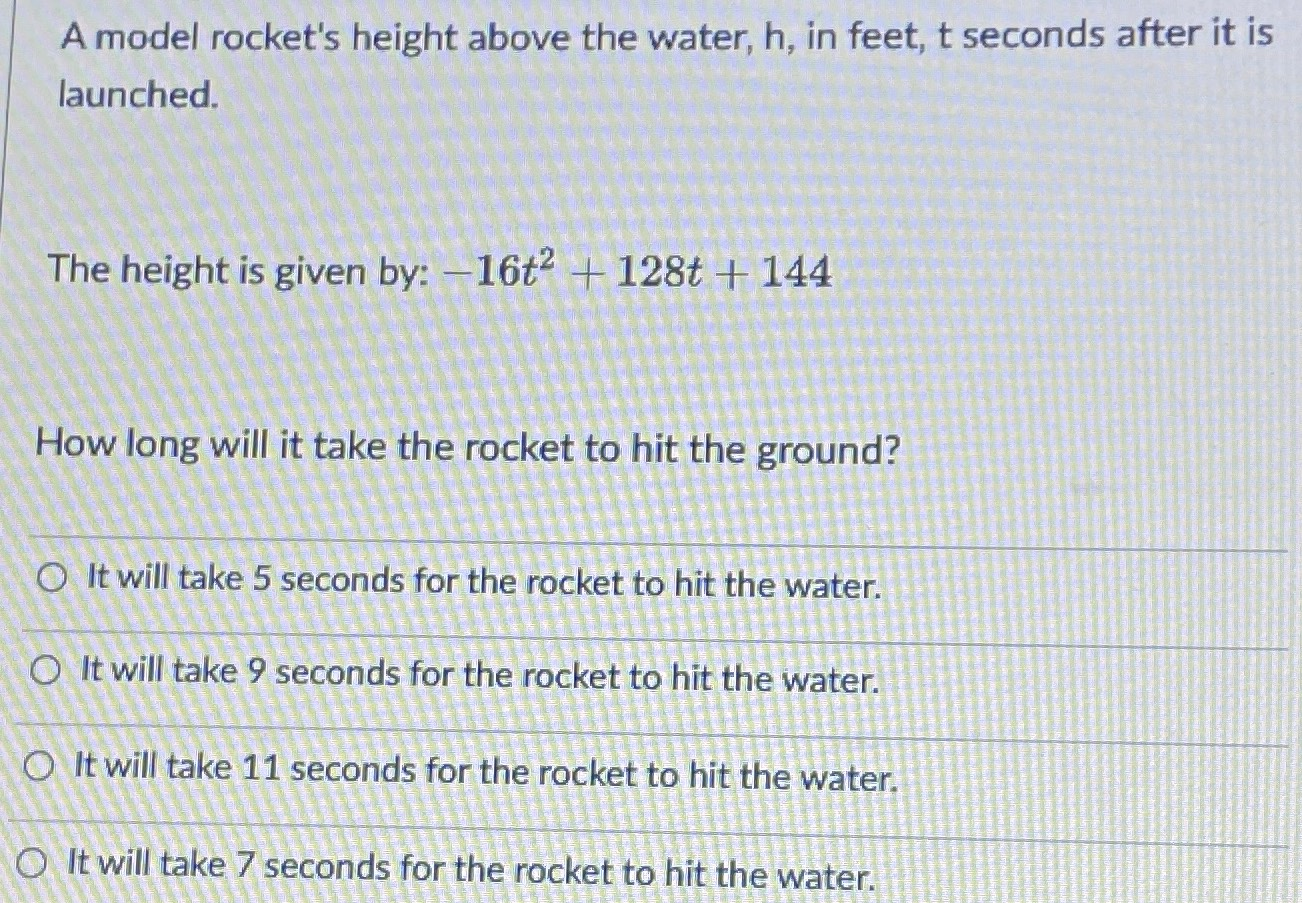### Still have math questions?

Algebra
QuestionA model rocket's height above the water, $$h$$ , in feet, $$t$$ seconds after it is launched.

The height is given by: $$- 16 t ^ { 2 } + 128 t + 144$$

How long will it take the rocket to hit the ground?

A. It will take $$5$$ seconds for the rocket to hit the water.

B. It will take $$9$$ seconds for the rocket to hit the water.

C. It will take $$11$$ seconds for the rocket to hit the water.

D. It will take $$7$$ seconds for the rocket to hit the water.